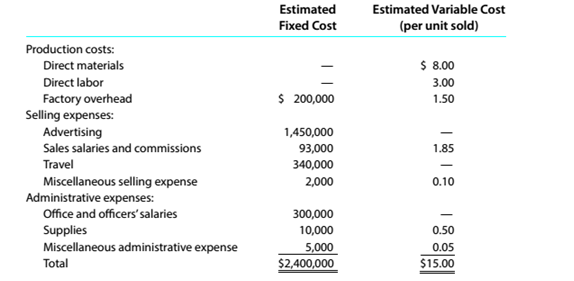Chapter 11, Problem 11.6.2P

Chapter
Section
Textbook Problem

Contribution margin, break-even sales, cost-volume-profit graph, and operating leverage Organic Health Care Products Inc. expects to maintain the same inventories at the end of 20Y8 as at the beginning of the year. The total of all production costs for the year is therefore assumed to be equal to the cost of goods sold. With this in mind, the various department heads were asked to submit estimates of the costs for their departments during 20Y8. A summary report of these estimates is as follows:It is expected that 400.000 units will be sold at a price of \$25 a unit. Maximum sales within the relevant range are 500.000 units.InstructionsWhat is the expected contribution margin ratio?

To determine

Concept Introduction:

Cost Volume Profit (CVP) Analysis:

The Cost Volume Profit analysis is the analysis of the relation between cost, volume, and profit of a product. It analyzes the cost and profits at the different level of production, in order to determine the breakeven point and required the level of sales to earn the desired profit.

Contribution margin means the margin that is left with the company after recovering variable cost out of revenue earned by selling smart phones. The formula for contribution margin is as follows:

Contribution margin = Sales - Variable cost.

Similarly contribution margin ratio = Contribution/sales

To Calculate:

The Expected Contribution Margin ratio

Explanation

The Expected Contribution Margin ratio is calculated as follows;

 Selling Price per unit (A) \$ 25 Variable Cost per unit (B)

Still sussing out bartleby?

Check out a sample textbook solution.

See a sample solution

The Solution to Your Study Problems

Bartleby provides explanations to thousands of textbook problems written by our experts, many with advanced degrees!

Get Started

Find more solutions based on key concepts